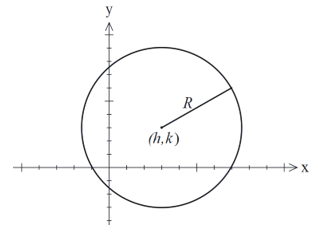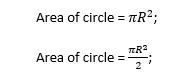# Equation of semicircle (Upper and Lower) - Formula, Examples

Home > Equation of Semicircle

Page Contents

## What is the Equation of Semicircle?

The equation of a semicircle can be deduced from the equation of a circle. A semicircle is simply half of a circle. There are two types of semicircle equations – Upper semicircle and lower semicircle equation.

semicircle is formed when a lining passing through the center touches the two ends of the circleIn the above figure, line AB is called the diameter of the circle. The diameter divides the circle into two halves such that they are equal in area. These two halves are referred to as the semicircle.

Equation of a semi-circle at the origin: $y = \pm \sqrt{R^{2} – x^{2}}$

So, Equation of upper semicircle at origin: $y = + \sqrt{R^{2} – x^{2}}$

Equation of lower semicircle at origin: $y = – \sqrt{R^{2} – x^{2}}$

Before understanding the Equation of semicircles, let’s discuss the circle first.

The set of all the points in a plane, which are at a constant distance from a fixed point or a center or an origin in the plane, is called a circle. The constant distance is called as Radius (R) of a circle and the fixed point is called the origin (O) or center of a circle.Example: A coin, Clock, Ring, Wheel, and Disc.

When a circle cuts into two equal parts along a diameter it is formed a semicircle. The following diagram represents two semicircles (upper and lower) along with the one symmetry line which is called Diameter (D = 2R). The line AB is the one symmetry line which is called reflection symmetry as well.If AB is not equal to the diameter of the circle then it forms two arcs on the following condition:## Equation of Semicircle at the origin

We know that the equation of the circle is:

$(x-h)^2+ (y-k)^2= R^2$Where (h, k) is the coordinate of the center of the circle and R is the radius of the circle.

A circle is divided into lower and upper semicircles. Let the coordinate of a center be (0, 0) and the radius of a circle is R.

So, h = 0, and K = 0;

Equation of circle is:

$(x-0)^2+ (y-0)^2=R^2$

$x^2+ y^2=R^2$

$y^2=R^2-x^2$

## Equation of Semicircle at the center (h, k)

Let, the co-ordinate of a center of a circle is (2, 3) and the radius is 3:

So the equation of the circle is:A circle is divided into lower and upper semicircles. The coordinate of a center is (2, 3) and the radius of a circle is 3.

So, h = 2, and K = 3;

Equation of circle is:

$(x-2)^2+ (y-3)^2=3^2$

$(x-2)^2+ (y-3)^2=9$

$(y-3)^2=9 – (x-2)^2$

$y = \pm \sqrt{9 – (x – 2)^{2}} + 3$

So, Equation of upper semicircle: $y = + \sqrt{9 – (x – 2)^{2}} + 3$

Equation of lower semicircle: $y = – \sqrt{9 – (x – 2)^{2}} + 3$

## How to find the equation of semicircle (upper and lower).

Example 1: Let the radius of a circle be 2 and the coordinate of the center of the circle is the origin i. e. (0,0).

Solution: Putting h = 0, and k = 0, and radius, R =2 in the equation

$(x-h)^2+ (y-k)^2=R^2$

So, we get

$(x-0)^2+ (y-0)^2=2^2$

$(x)^2+ (y)^2=4$

Equation of upper semicircle = $y = + \sqrt{4 – x^{2}}$

Equation of lower semicircle = $y = – \sqrt{4 – x^{2}}$

Example 2: What is the equation of lower and upper semicircles if the coordinate of the circle is (2, 0) and the radius of the circle is 3?

Solution: Putting h = 2, and k = 0, and radius, R =3 in the equation

$(x-h)^2+ (y-k)^2=R^2$

We get,

$(x-2)^2+ (y-0)^2 = 3^2$

$(x-2)^2+ (y)^2 = 9$

$y^2= 9 – (x-2)^2$

$y = \pm \sqrt{9 – (x – 2)^{2}}$

So, Equation of upper semicircle: $y = + \sqrt{9 – (x – 2)^{2}}$

Equation of lower semicircle: $y = – \sqrt{9 – (x – 2)^{2}}$

Example 3: What is the equation of lower and upper semicircle if the co-ordinate of circle is (0, 4) and radius of the circle is 5?

Solution: Putting h = 0, and k = 4, and radius, R = 5 in equation

$(x-h)^2+ (y-k)^2=R^2$

so, we get

$(x-0)^2+ (y-4)^2=5^2$

$(x-0)^2+ (y-4)^2=25$

$(y-4)^2=25 – x^2$

$y – 4 = ± \sqrt{25 – x^{2}}$

$y = 4 ± \sqrt{25 – x^{2}}$

Equation of upper semicircle: $y = 4 + \sqrt{25 – x^{2}}$

Equation of lower semicircle: $y = 4 – \sqrt{25 – x^{2}}$

Example 4: What is the equation of lower and upper semicircles if the coordinate of the circle is (3, 4) and the radius of the circle is 6?

Solution: Putting h = 3, and k = 4, and radius, R = 6 in equation,

⇒ (x – h)2 + (y – k)² = R²

So, we get

(x – 3)2 + (y – 4)² = 6²

(x – 3)2 + (y – 4)² = 36

(y – 4)² = 36 – (x – 3)2

$y – 4 = ± \sqrt{36 – (x-3)^{2}}$

Equation of upper semicircle: $y = 4 + \sqrt{36 – (x-3)^{2}}$

Equation of lower semicircle: $y = 4 – \sqrt{36 – (x-3)^{2}}$

## The Calculation for the equation of Area and Perimeter of a semicircleArea: Area is the size that expresses the coverage of a two-dimensional shape in the plane.

The area of a circle refers to the inner space of the circle. As we know a semicircle is formed by cutting a circle into two equal parts along with diameter. So the area of the semicircle will be half of the area of the circle. Where radius R is constant for both circle and semicircle.## How to find the equation of the area of a semicircle:

For a circle, the radius R (OA) varies from 0 (zero) to ∞ (infinity). If OA is rotated anticlockwise from 0 degrees to 180 degrees then reached to B. So, it is formed a semicircle.

To find the area of a semicircle, we need to use the cylindrical coordinate system and use the surface integration.

The coordinates of cylindrical coordinate systems are (R, θ, Ζ)

Where R varies from 0 (zero) to ∞ (infinity), θ varies from 0 degrees to 180 degrees, and Z is constant.## Equation of perimeter of Semicircle

A perimeter of a semicircle is the closed path that surrounds the outer line. It is also defined as the one-dimensional length of any two- dimensional shape.

As we know, the semicircle is half of a circle so it does not mean that the perimeter is also half of a circle.

⇒ We know that, the perimeter of the circle = 2πR

⇒ The perimeter of semicircle ≠ 2πR

Where R is the radius of the circle or semi-circle

As per the definition of the perimeter, it is the sum of the arc length and the diameter of the semicircle.

So, Perimeter of circle = Length of arc AB + Diameter;

Length of arc, AB = 2πR(θ/360º)

Here, θ = 180º

Length of arc, AB = 2πR(180º/360º) = πR;  [∴ θ = 180º]

Diameter (D) = πR

The perimeter of semicircle = (πR + D) or (πR + 2R);

The equation of Perimeter of semicircle = R(π+2)

Also check –

Share it...